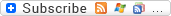Passover / Pesach Cooking Conversion Calculator - Check out what those weights and measurements are! Convert from imperial to metric and vice-versa!

After you're done with this page, do you want to find out what all those cooking abbreviations are that you see in recipes? Head on over to our Passover / Pesach Cooking Abbreviations page to find out! Now, it's on to calculating those weights and measures!

Conversion of Oven Temperatures
FahrenheitCelsiusFahrenheitCelsius
100 degrees F38 degrees C212 degrees F100 degrees C
110 degrees F43 degrees C225 degrees F110 degrees C
115 degrees F46 degrees C250 degrees F120 degrees C
135 degrees F57 degrees C275 degrees F135 degrees C
140 degrees F60 degrees C300 degrees F150 degrees C
150 degrees F66 degrees C325 degrees F165 degrees C
160 degrees F71 degrees C350 degrees F180 degrees C
165 degrees F74 degrees C375 degrees F190 degrees C
170 degrees F77 degrees C400 degrees F205 degrees C
180 degrees F82 degrees C425 degrees F220 degrees C
190 degrees F88 degrees C450 degrees F230 degrees C
200 degrees F95 degrees C475 degrees F245 degrees C
205 degrees F96 degrees C500 degrees F260 degrees C

OVEN TEMPERATURES
Very Slow250F to 300F
Slow300F to 325F
Moderate350F to 375F
Hot400F to 425F
Very Hot450F to 475F

Or, just use the temperature conversion calculator below!

Just enter a value in one box and then click outside the boxes to get the other three temperatures.

Some Unusual Weights and Measurements
1 bit = 2 pinches
1 dollop = 2 smidgens (2 rounded tablespoons)
1 gaggle = 3 dollops
1 gill = 2/3 of a cup (or a little less)
1 jigger = 1-1/2 Fluid Ounces
1 pinch = the amount between the tip of one's finger and thumb
1 pony = 1 Fluid Ounce
1 smidgen = 4 bit (or 1 rounded tablespoon)
1 speck = less than 1/8 of a teaspoon
A few grains = less than 1/8 of a teaspoon

Miscellaneous Measurements
juice of 1 lemon = 2 to 3 tablespoons
juice of 1 orange = about 1/2 cup

Want more cooking conversions? Check out the tables below:

## U.S. and Metric Measurement Charts

U.S. Measurement Equivalents

 A few grains/pinch/dash, (dry) = Less than 1/8 teaspoon A dash (liquid) = A few drops 3 teaspoons = 1 tablespoon 1/2 tablespoon = 1 1/2 teaspoons 1 tablespoon = 3 teaspoons 2 tablespoons = 1 fluid ounce 4 tablespoons = 1/4 cup 5 1/3 tablespoons = 1/3 cup 8 tablespoons = 1/2 cup 8 tablespoons = 4 fluid ounces 10 2/3 tablespoons = 2/3 cup 12 tablespoons = 3/4 cup 16 tablespoons = 1 cup 16 tablespoons = 8 fluid ounces 1/8 cup = 2 tablespoons 1/4 cup = 4 tablespoons 1/4 cup = 2 fluid ounces 1/3 cup = 5 tablespoons plus 1 teaspoon 1/2 cup = 8 tablespoons 1 cup = 16 tablespoons 1 cup = 8 fluid ounces 1 cup = 1/2 pint 2 cups = 1 pint 2 pints = 1 quart 4 quarts (liquid) = 1 gallon 8 quarts (dry) = 1 peck 4 pecks (dry) = 1 bushel 1 kilogram = approximately 2 pounds 1 liter = approximately 4 cups or 1 quart

Approximate Metric Equivalents by Volume
U.S.    Metric
1/4 cup = 60 milliliters
1/2 cup = 120 milliliters
1 cup = 230 milliliters
1 1/4 cups = 300 milliliters
1 1/2 cups = 360 milliliters
2 cups = 460 milliliters
2 1/2 cups = 600 milliliters
3 cups = 700 milliliters
4 cups (1 quart) = .95 liter
1.06 quarts = 1 liter
4 quarts (1 gallon) = 3.8 liters

Approximate Metric Equivalents by Weight
U.S.   Metric
1/4 ounce = 7 grams
1/2 ounce = 14 grams
1 ounce = 28 grams
1 1/4 ounces =  35 grams
1 1/2 ounces = 40 grams
2 1/2 ounces = 70 grams
4 ounces = 112 grams
5 ounces = 140 grams
8 ounces = 228 grams
10 ounces = 280 grams
15 ounces = 425 grams
16 ounces (1 pound) = 454 grams